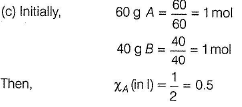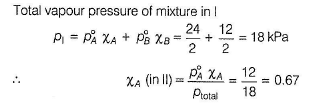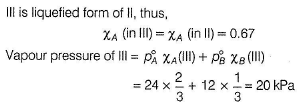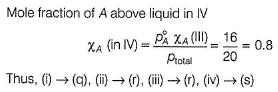Courses

# Test: Colligative Properties I (Lowering Of Vapour Pressure)

## 20 Questions MCQ Test Chemistry Class 12 | Test: Colligative Properties I (Lowering Of Vapour Pressure)

Description
This mock test of Test: Colligative Properties I (Lowering Of Vapour Pressure) for Class 12 helps you for every Class 12 entrance exam. This contains 20 Multiple Choice Questions for Class 12 Test: Colligative Properties I (Lowering Of Vapour Pressure) (mcq) to study with solutions a complete question bank. The solved questions answers in this Test: Colligative Properties I (Lowering Of Vapour Pressure) quiz give you a good mix of easy questions and tough questions. Class 12 students definitely take this Test: Colligative Properties I (Lowering Of Vapour Pressure) exercise for a better result in the exam. You can find other Test: Colligative Properties I (Lowering Of Vapour Pressure) extra questions, long questions & short questions for Class 12 on EduRev as well by searching above.
QUESTION: 1

### This section contains 12 multiple choice questions. Each question has four choices (a), (b), (c) and (d), out of which ONLY ONE is correct The vapour pressure of pure liquid solvent is 0.80 atm. When a non-volatile solute B is added to the solvent, its vapour pressure drops to 0.60 atm. Thus, mole fraction of the component B is

Solution:

Using Raoults law for lowering of vapour pressure.We get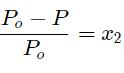putting,Values of actual and lowered vapour pressure we get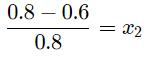where x2​ is the mole fraction .

QUESTION: 2

### Vapour pressure of pure water at 298 K is 23.8 torr. Thus, vapour pressure of a solution containing 6 g urea and 68.4 g sucrose in 10 moles of water is

Solution:

By Raoult’s law, for a mixture of non-volatile solute in volatile solvent.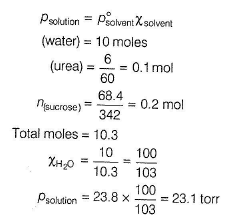QUESTION: 3

### Vapour pressure of nitrobenzene (molar mass = 123 g mol-1) is 3.6 kNm-2 and that of water (molar mass = 18 g mol-1) is 977 kNm-2. They form immiscible mixture at the given temperature. Thus, percentage of nitrobenzene in the vapour phase is

Solution:

For immiscible solvents, composition of each component is based on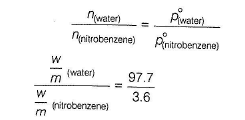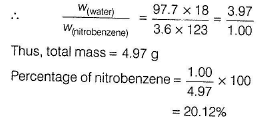QUESTION: 4

The vapour pressure of n-hexane at 350 K is 840 torr and that of cyclohexane is 600 torr. Mole fraction of hexane in the mixture that boils at 350 K and 1 atm pressure assuming ideal behaviour is

Solution:

d) Mixture boils at 1 atm and 350 K.
Thus, total vapour pressure is 1 atm = 760torr (h) used for hexane and (ch) for cyclohexane.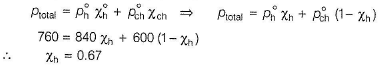QUESTION: 5

At 298 K, the vapour pressure of pure water is 23.76 mm Hg and that of an aqueous solution is 22.98 mm Hg. Thus, molality of solution is (assume dilute solution)

Solution:

(c)

By Raoult’s law for a non-volatile solute in volatile solvent.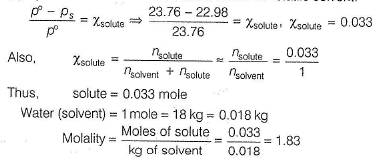QUESTION: 6

A binary liquid solution is prepared by mixing n-heptane and ethanol. Which one of the following statements is/are correct regarding the behaviour of the solution?

Solution:

Ethanol molecules are joined to each other by intermolecular H-bonding thus, they have high boiling point. When n-hexane is mixed into it, H-bonding breaks. Since, force of attraction between n-hexane and ethanol molecules is smaller than between the hexane-hexane and ethanol-ethanol. Thus, mixture is more volatile. This shows positive deviation from Raoult’s law

QUESTION: 7

18 g of glucose (C6H12O6 ) is added to 178.2 g of water. The vapour pressure of water for this aqueous solution at 100°C is

Solution:

(d)

At 100°C, vapour pressure of water = 1 atm. Thus, p0 = 760torr Let vapour pressure of the solution = ps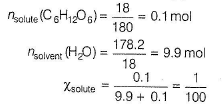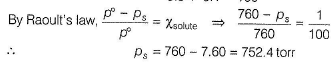QUESTION: 8

Which of the following liquid pairs shows a positive deviation from Raoult’s law?

Solution:

(a) When HCI is mixed with H20, force of attraction between HCI and H2O  molecules is increased (due to dissolution), hence vaporisation decreased, a case of negative deviation.

(b) CH3OH molecules are joined by intermolecular H-bonding which provides it a liquid state. On adding benzene, H-bonding breaks and force of attraction between CH3OH and benzene molecules is less than between CH3OH-CH3OH and between benzene-benzene hence, more vaporisation of mixture, a case of positive deviation.

(c) As in (a), negative deviation.

(d) CH3COCH3 and CHCI3 are volatile. On mixing, there is increase in intermolecular H-bonding due to the formation of chloretone (A)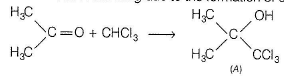Thus, force of attraction between (CHCI3-CH3OCH3) molecules is larger than between (CHCI3-CHCI3)and (CH3COCH3 - CH3OC H3) molecules. Thus, vaporisation decreases, a case of negative deviation.

QUESTION: 9

A solution of two liquids boils at a temperature more than the boiling point of either of them. Hence, the binary solution shows

Solution:

If force of attraction between A and B (solvents) is higher than that between A and A or B and B, then there is decrease in vaporisation.
Thus, higher temperature (i.e. boiling point) is required to convert liquids into vapour state. This is a case of negative deviation from Raoult's law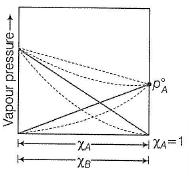QUESTION: 10

Lowering of vapour pressure of an aqueous solution of a non-volatile non-electrolyte 1 molal aqueous solution at 100°C is

Solution:

(c) Molality = 1 mol kg-1 (solvent) Thus, solute = 1 mole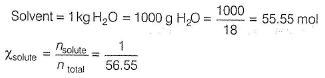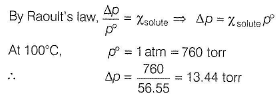QUESTION: 11

Which combination of following terms is matched correctly?
I. Vapour pressure

II. Intermolecular forces

III. Latent heat of vaporisation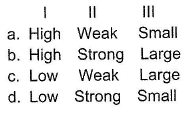Solution:

(a) Liquid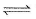Vapour

(a) If vapour pressure is high, it means equilibrium is in forward side. This is attained when intermolecular forces holding the molecules are of weak nature. Low heat is required to convert liquid into vapour. Thus, (a) is correct.

(b) Thus, incorrect.

(c) When vapour pressure is low, it means intermolecular forces are strong hence (c) is incorrect.

(d) When vapour pressure is low, intermolecular forces are strong, hence large heat is required thus, (d) is incorrect,

QUESTION: 12

The solubility of urea in methanol is 17 g urea/100 mL methanol. Density of methanol is 0.792 g/mL at 293 K at which vapour pressure of methanol is 96.0 mm of Hg. Thus, vapour pressure of saturated solution of urea in methanol is

Solution: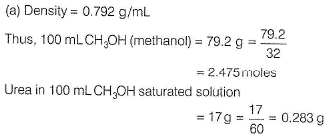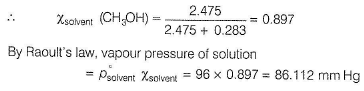*Multiple options can be correct
QUESTION: 13

One or More than One Options Correct Type
This section contains 3 multiple type questions. Each question has 4 choices (a), (b), (c) and (d), out of which ONE or MORE THAN ONE are correct.

Q. Benzene and naphthalene form an ideal solution at room temperature. For this process, the true statement(s) is/are  [JEE Advanced 2013]

Solution:

When binary mixture is ideal, then by Raoult’s law

ΔV = 0, ΔHmix = 0, no enthalpy change

Mixing is spontaneous, thus ΔG < 0 By thermodynamic property,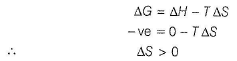Thus, on mixing, entropy increases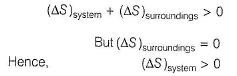*Multiple options can be correct
QUESTION: 14

Total vapour pressure of mixture of 1 mole of a volatile component A (p°A = 100 mm Hg) and 3 moles of volatile component B(p°B = 60 mm Hg) is 75 mm Hg. For such case,

Solution: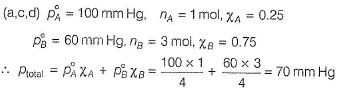But, actual vapour pressure = 75 mm Hg. Vapour pressure is increased. Force of attraction between A and B is smaller than between A and A and between B and B. Thus, more vaporisation due to decrease in boiling point, a case of positive deviation.

QUESTION: 15

At 35°C, the vapour pressure of carbon disulphide (CS2)is 512 mm Hg and that of acetone (CH3COCH3)is 344 mm Hg. A solution of CS2 and CH3COCH3 in which mole fraction of CS2 is 0.25 has a total vapour pressure of 600 mm Hg. Which of the following statement(s) is/are correct?

Solution:

(A for acetone, C for carbon disulphide)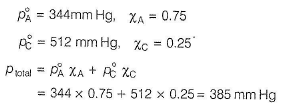But, actual ptotal = 600 mm Hg

Thus, there is positive deviation. Boiling point is lowered.
Thus, ΔH = + ve (endothermic), i.e. heat is absorbed.

QUESTION: 16

Comprehension Type
This section contains 2 paragraphs , each describing theory, experiments, data, etc. four questions related to the paragraph have been given. Each question has only one correct answer out of the given 4 options (a), (b), (c) and (d)

Passage I
At 300 K, vapour pressure of pure liquid A = 575torr
and that of pure liquid B = 390torr
In binary mixture of liquids A and B with ideal behaviour, mole fraction of A in vapour phase =0.35
Q. Binary mixture contains

Solution:

Let moles of A = nA and moles of B = nB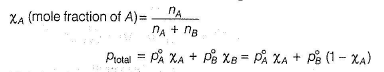Mole fraction of A in vapour state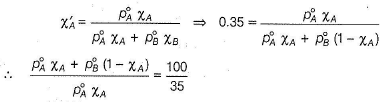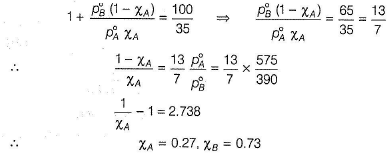Thus, A is 27 mole %.
Total vapour pressure of binary mixture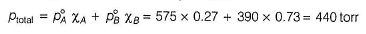QUESTION: 17

Passage I

At 300 K, vapour pressure of pure liquid A = 575torr and that of pure liquid B = 390torr In binary mixture of liquids A and B with ideal behaviour, mole fraction of A in vapour phase =0.35

Q. Total vapour pressure of the binary mixture is

Solution:

Let moles of A = nA and moles of B = nBMole fraction of A in vapour stateThus, A is 27 mole %.
Total vapour pressure of binary mixtureQUESTION: 18

Passage II

At 40°C, the vapour pressure (in torr) of methanol-ethanol solution is represented by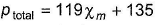where,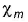is the mole fraction of methanol.

Q. Difference of vapour pressures of volatile liquids (methanol-ethanol) in pure state at 40°C is

Solution: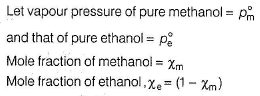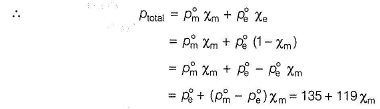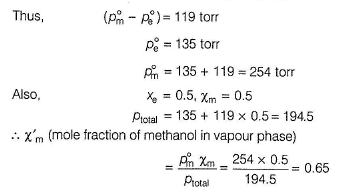QUESTION: 19

Passage II

At 40°C, the vapour pressure (in torr) of methanol-ethanol solution is represented bywhere,is the mole fraction of methanol.

Q.

What is the mole fraction of methanol in vapour phase in a binary mixture with mole fraction of ethanol 0.50?

Solution:

(C)QUESTION: 20

Matching List Type
Choices for the correct combination of elements from Column I and Column II are given as options (a), (b), (c) and (d), out of which one is correct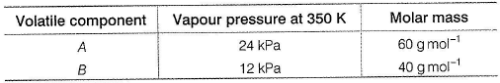100 g of binary mixture of A and B contains 60% of A. Mixture is subjected to the following operation.

Original mixture I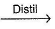II containing vapours of A and B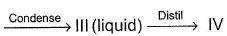containing vapours of A and B

Match the content of Column I with their values in Column II and select the answer from the codes given below.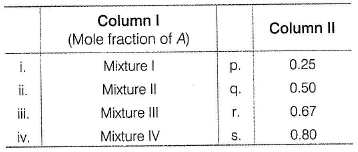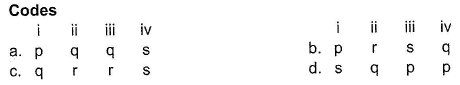Solution: